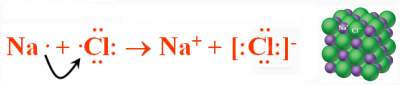# Ionic Bonding

## Introduction

Cations and anions are formed in such a way that the "OctetRule" is satisfied.
Example:
Na(s) + ½Cl2 → NaCl(s)
ΔH°r = − 410.9 Kj (highly exothermic)
Both Na and Cl in NaCl have inert gas configuration.### Energetics of Ionic Bond Formation

Definition: Lattice energy is the energy required to completely separate an ionic solid into its gaseous ions. E1 = κ(Q1Q2)/d
Where: κ is a constant = 8.99 x 109 J·m/C2, Q1 and Q2 are the charges on the ions, d is the distance between ions.

Lattice energy depends on:
• Charges on the ions (Q1 and Q2)
• Sizes of the ions (d)
Lattice energy increases as:
• Charges on the ions increase
• The distance between the ions decreases

### Exercise: Energetics of Ionic Bond Formation

Exercise on Energetics of Ionic Bond Formation

Check your answers here: Solution to the Exercise on Energetics of Ionic Bond Formation

For more details, please contact me here.
Date of last modification: Summer , 2019